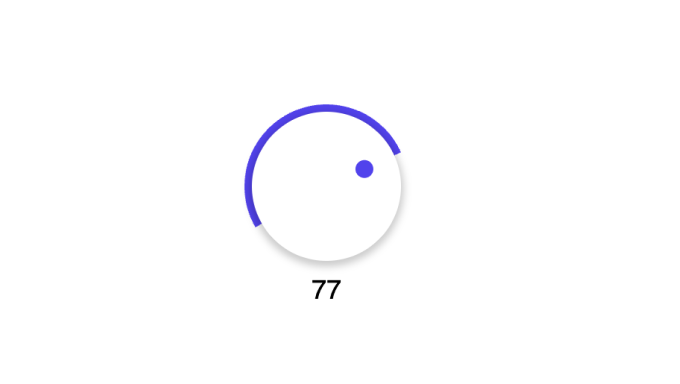# Rotary Knob

This is an example of how to use the Script node to implement a simple rotary knob.

The knob has start and stop angles, and is not "endless".## Inputs​

Min Value The minimum value the component should output

Max Value The maximum value the component should output

Value Set the start value, must be between Min Value and Max Value

Min Angle The lowest angle the knob can turn to. Should be between -180 and 180.

Max Angle The maximum angle the knob can turn to. Should be between -180 and 180.

## Outputs​

Value The current value